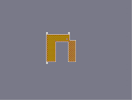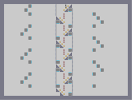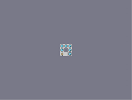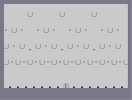### oraNgeHover over the thumbnail for a full-size version.

Author killn2 author:killn2 n-art nonplayable orange rated 2008-02-27 2 by 5 people. \$oraNge#killn2#none#111111111111111111111111111111111111111111111111111111111111111111111111111111111111111111111111111111111111111111111111111111111111111111111111111111111111111111111111111111111111111111111111111111111111111111111111111111111111111111111QQQQQQQQ111111111111111P000000N111111111111111P000000N111111111111111P0N11111111111111111111P0N11111111111111111111P0N11111111111111111111OO00000N1111111111111111100000N1111111111111111111111111111111111111111111111111111111111111111111111111111111111111111111111111111111111111111111111111111111111111111111111111111111111111111111111111111111111111111111111111111111111111111111111111111111111111111111111111111111111111111111111111111111111111111111111111111111111111111111|0^282,366!0^282,354!0^282,342!0^282,330!0^282,318!0^282,306!0^282,294!0^282,282!0^282,270!0^282,258!0^282,246!0^282,234!0^282,222!0^282,210!0^294,210!0^306,210!0^318,210!0^330,210!0^342,210!0^354,210!0^366,210!0^378,210!0^390,210!0^402,210!0^414,210!0^414,222!0^414,234!0^414,246!0^402,246!0^390,246!0^378,246!0^366,246!0^354,246!0^342,246!0^330,246!0^330,258!0^330,270!0^330,282!0^330,294!0^330,306!0^330,318!0^330,330!0^330,342!0^330,354!0^330,366!0^318,366!0^306,366!0^294,366!0^414,258!0^414,270!0^414,282!0^414,294!0^414,306!0^414,318!0^414,330!0^414,342!0^414,354!0^414,366!0^426,366!0^438,366!0^450,366!0^450,354!0^450,342!0^450,330!0^450,318!0^450,306!0^450,294!0^450,282!0^450,270!0^450,258!0^450,246!0^438,246!0^426,246!0^420,252!0^432,252!0^444,252!0^444,264!0^438,258!0^426,258!0^420,264!0^432,264!0^426,270!0^438,270!0^444,276!0^432,276!0^420,276!0^426,282!0^438,282!0^444,288!0^432,288!0^420,288!0^420,300!0^426,294!0^432,300!0^438,294!0^444,300!0^426,306!0^438,306!0^444,312!0^432,312!0^420,312!0^426,318!0^438,318!0^444,324!0^432,324!0^420,324!0^426,330!0^438,330!0^444,336!0^432,336!0^420,336!0^426,342!0^438,342!0^444,348!0^432,348!0^420,348!0^426,354!0^438,354!0^444,360!0^432,360!0^420,360!12^414,360!12^414,348!12^414,336!12^414,324!12^414,312!12^414,300!12^414,288!12^414,276!12^414,264!12^414,252!12^420,246!12^432,246!12^444,246!12^450,252!12^450,264!12^450,276!12^450,288!12^450,300!12^450,312!12^450,324!12^450,336!12^450,348!12^450,360!12^444,366!12^432,366!12^420,366!12^420,354!12^432,354!12^426,360!12^438,360!12^444,354!12^438,348!12^426,348!12^420,342!12^432,342!12^444,342!12^438,336!12^426,336!12^420,330!12^432,330!12^444,330!12^438,324!12^426,324!12^420,318!12^432,318!12^444,318!12^438,312!12^426,312!12^420,306!12^432,306!12^444,306!12^438,300!12^426,300!12^420,294!12^432,294!12^444,294!12^438,288!12^426,288!12^420,282!12^432,282!12^444,282!12^438,276!12^426,276!12^420,270!12^432,270!12^444,270!12^438,264!12^426,264!12^420,258!12^432,258!12^444,258!12^438,252!12^426,252!12^414,240!12^414,228!12^414,216!12^408,210!12^396,210!12^384,210!12^372,210!12^360,210!12^348,210!12^336,210!12^324,210!12^312,210!12^300,210!12^288,210!12^282,216!12^282,228!12^282,240!12^282,252!12^282,264!12^282,276!12^282,288!12^282,300!12^282,312!12^282,324!12^282,336!12^282,348!12^282,360!12^288,366!12^300,366!12^312,366!12^324,366!12^330,360!12^330,348!12^330,336!12^330,324!12^330,312!12^330,300!12^330,288!12^330,276!12^330,264!12^330,252!12^336,246!12^348,246!12^360,246!12^360,246!12^372,246!12^384,246!12^396,246!12^408,246!12^324,354!12^312,354!12^300,354!12^288,354!12^294,360!12^306,360!12^318,360!12^318,348!12^306,348!12^294,348!12^288,342!12^300,342!12^312,342!12^324,342!12^318,336!12^306,336!12^294,336!12^288,330!12^300,330!12^312,330!12^324,330!12^318,324!12^306,324!12^294,324!12^288,318!12^300,318!12^312,318!12^324,318!12^318,312!12^306,312!12^294,312!12^288,306!12^300,306!12^312,306!12^324,306!12^318,300!12^306,300!12^294,300!12^288,294!12^300,294!12^312,294!12^324,294!12^318,288!12^306,288!12^294,288!12^288,282!12^300,282!12^312,282!12^324,282!12^318,276!12^306,276!12^294,276!12^288,270!12^300,270!12^312,270!12^324,270!12^318,264!12^306,264!12^294,264!12^288,258!12^300,258!12^312,258!12^324,258!12^318,252!12^324,246!12^312,246!12^306,240!12^300,234!12^294,228!12^288,222!12^294,216!12^300,222!12^306,228!12^312,234!12^318,240!12^324,246!12^306,252!12^300,246!12^294,240!12^288,234!12^288,246!12^294,252!12^306,216!12^312,222!12^324,234!12^330,240!12^318,216!12^324,222!12^330,228!12^336,234!12^348,234!12^342,228!12^330,216!12^336,222!12^342,240!12^354,240!12^366,240!12^378,240!12^390,240!12^402,240!12^408,234!12^396,234!12^384,234!12^372,234!12^360,234!12^354,228!12^348,222!12^342,216!12^354,216!12^366,216!12^378,216!12^390,216!12^402,216!12^408,222!12^402,228!12^396,222!12^390,228!12^384,222!12^378,228!12^372,222!12^366,228!12^360,222!0^324,360!0^312,360!0^300,360!0^288,360!0^288,348!0^294,354!0^300,348!0^306,354!0^312,348!0^318,354!0^324,348!0^318,342!0^306,342!0^294,342!0^288,336!0^300,336!0^312,336!0^324,336!0^318,330!0^306,330!0^294,330!0^288,324!0^300,324!0^312,324!0^324,324!0^318,318!0^306,318!0^294,318!0^288,312!0^300,312!0^312,312!0^324,312!0^318,306!0^306,306!0^294,306!0^288,300!0^294,294!0^300,300!0^306,294!0^312,300!0^318,294!0^324,300!0^324,288!0^312,288!0^300,288!0^288,288!0^294,282!0^306,282!0^318,282!0^324,276!0^312,276!0^300,276!0^288,276!0^294,270!0^306,270!0^318,270!0^324,264!0^312,264!0^300,264!0^288,264!0^294,258!0^306,258!0^318,258!0^324,252!0^312,252!0^300,252!0^288,252!0^318,246!0^306,246!0^294,246!0^288,240!0^294,234!0^288,228!0^288,216!0^300,216!0^294,222!0^306,222!0^306,234!0^300,228!0^300,240!12^318,228!0^312,240!0^324,240!0^336,240!0^348,240!0^360,240!0^372,240!0^384,240!0^396,240!0^408,240!0^408,228!0^408,216!0^396,216!0^384,216!0^372,216!0^360,216!0^348,216!0^336,216!0^324,216!0^312,216!0^318,222!0^330,222!0^342,222!0^354,222!0^366,222!0^378,222!0^390,222!0^402,222!0^396,228!0^384,228!0^372,228!0^360,228!0^348,228!0^336,228!0^324,228!0^312,228!0^318,234!0^330,234!0^342,234!0^354,234!0^366,234!0^378,234!0^390,234!0^402,NaN# The idea just popped into my head

## Other maps by this authorA thwumpy tower E - Z Challenge 4 (tweeked) Nball

Pages: (0)

### you could have at least

tried to make the letter the same width everywhere. and what are those white things sticking up from it?

2/5 me thinks

### Actually...

I think killn2 was talking about using mines and gold together to create an orange color.

But that, too, has been around forever.

### Yer it pops into everyone's head

Everyone N-Artist does an N, whether its the little black dude made large like Dragon_moon's
http://numa.notdot.net/map/66137
or, like your's, the letter N written in Ned, naddict's
http://numa.notdot.net/map/25367
But hey it don't look bad just its not brilliant.